• Slides: 10Overview n n Hexadecimal (hex) ~ base 16 number system Use 0 through 9 and. . . A = 10 B = 11 C = 12 D = 13 E = 14 F = 15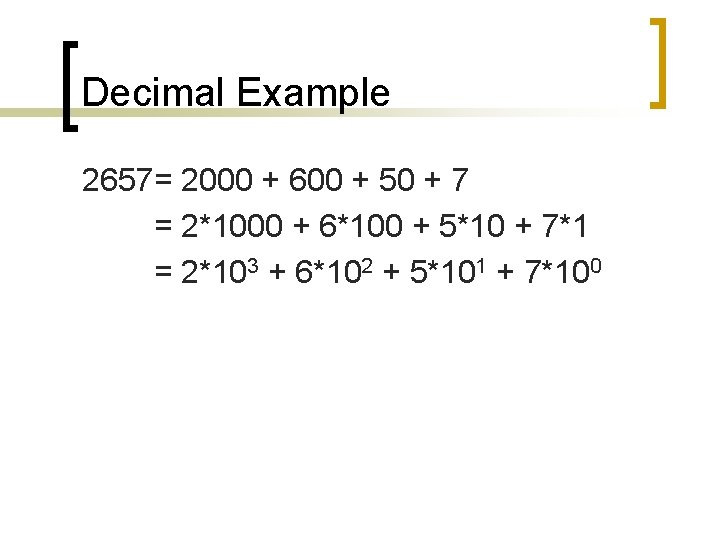Decimal Example 2657= 2000 + 600 + 50 + 7 = 2*1000 + 6*100 + 5*10 + 7*1 = 2*103 + 6*102 + 5*101 + 7*100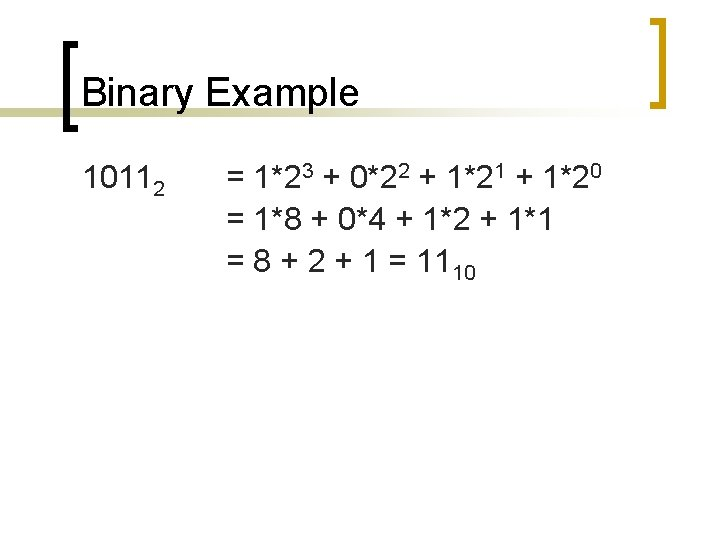Binary Example 10112 = 1*23 + 0*22 + 1*21 + 1*20 = 1*8 + 0*4 + 1*2 + 1*1 = 8 + 2 + 1 = 1110Hexadecimal Example A 4 F 16 = 10*162 + 4*161 + 15*160 = 10*256 + 4*16 + 15*1 = 2560 + 64 + 15 = 263910Hexadecimal Decimal 6116 = ? F 2316 = ? Now convert the above to binary. . .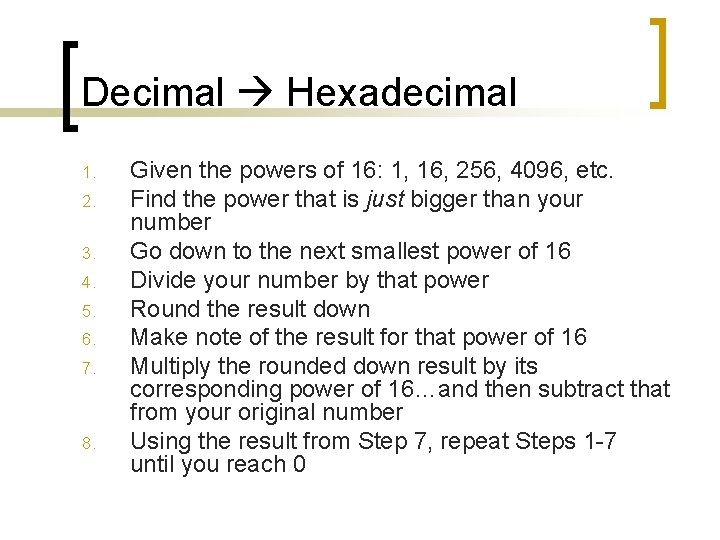Decimal Hexadecimal 1. 2. 3. 4. 5. 6. 7. 8. Given the powers of 16: 1, 16, 256, 4096, etc. Find the power that is just bigger than your number Go down to the next smallest power of 16 Divide your number by that power Round the result down Make note of the result for that power of 16 Multiply the rounded down result by its corresponding power of 16…and then subtract that from your original number Using the result from Step 7, repeat Steps 1 -7 until you reach 0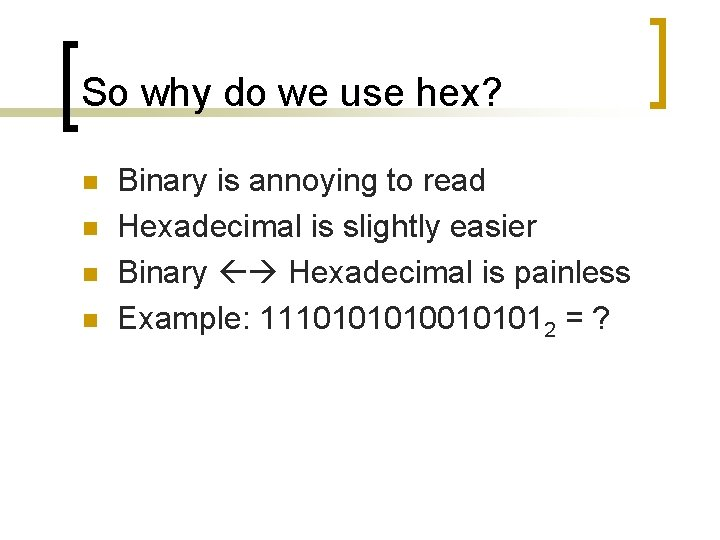So why do we use hex? n n Binary is annoying to read Hexadecimal is slightly easier Binary Hexadecimal is painless Example: 1110100101012 = ?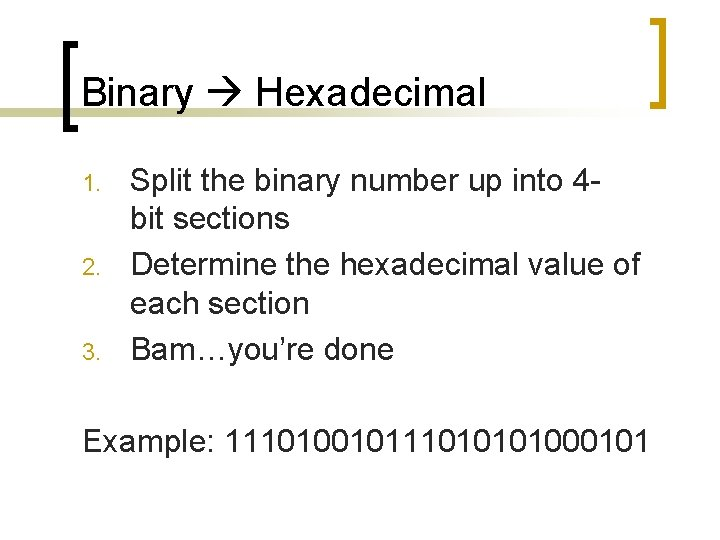Binary Hexadecimal 1. 2. 3. Split the binary number up into 4 bit sections Determine the hexadecimal value of each section Bam…you’re done Example: 11101001011101000101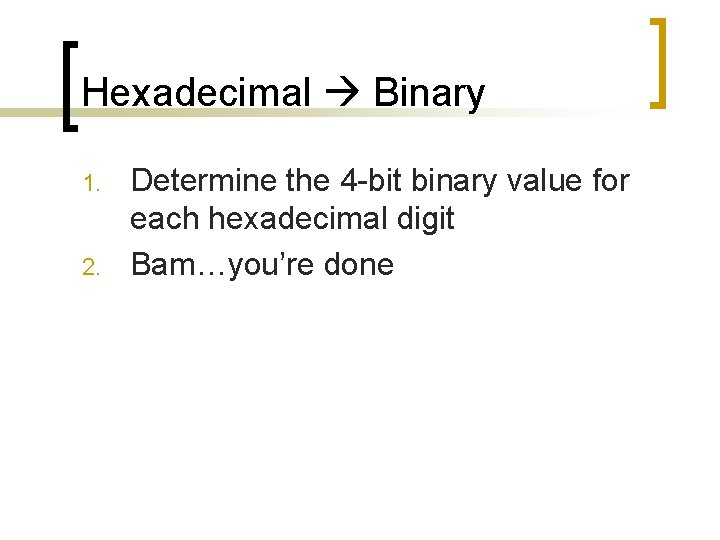Hexadecimal Binary 1. 2. Determine the 4 -bit binary value for each hexadecimal digit Bam…you’re done